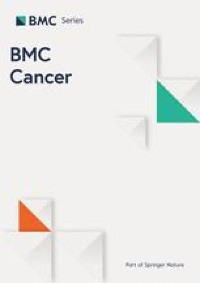# Reflecting on the utility of standardized uptake values on 18F-FDG PET in nasopharyngeal carcinoma – BMC Cancer

#### ByXiufang Qiu, Haixia Wu, Ting Xu, Shihan Xie, Ziqing You, Yixin Hu, Yinghong Zheng, Zewei Liang, Chaoxiong Huang, Li Yi, Li Li, Jing Liu, Zhaodong Fei and Chuanben Chen

May 5, 2022### Patients’ characteristics and outcomes

The characteristics of the patients are summarized in Supplementary Table S1. This study included a total of 369 patients with a median age of 47 years (range, 19–70 years). The median follow-up time was 51 months (range, 4–105 months). At the end of follow-up, 33 patients (8.9%) had died, and 48 patients (13%) had experienced disease relapse in the form of local recurrence (34 patients, 9.2%), regional failure (20 patients, 5.4%), and distant metastasis (47 patients, 12.7%). The 3-year LRFS, RRFS, DMFS, PFS, and OS rates were 93.2%, 96.2%, 88.9%, 81.3%, and 94.6%, respectively.

### SUVmax and clinical stage

The SUVmax-t and SUVmax-n increased with the T and N stage, respectively (Fig. 1). When the values were distributed according to the disease stage, we found that the SUVmax-t data were more concentrated than the SUVmax-n data. The median SUVmax-t for the T1, T2, T3, and T4 stages were 6.2, 7.7, 9.4, and 11.2, respectively. The median SUVmax-n for the N0, N1, N2, and N3 stages were 1.6, 5.6, 7.1, and 9.1, respectively. The SUVmax-t and SUVmax-n significantly differed between different T stages and between different N stages, respectively (P < 0.001, Fig. 1). However, the SUVmax-t was not significantly correlated with the SUVmax-n (r = 0.13, P < 0.001, Supplementary Figure S1).

### Optimal cutoff points for SUVmax-t and SUVmax-n

The mean SUVmax-t of the primary tumor and the mean SUVmax-n of cervical lymph node metastases were 9.2 ± 5.2 (range, 1.4–49.6) and 6.8 ± 5.8 (range, 0–36.6), respectively. The optimal cutoff SUVmax-t for predicting local recurrence was 7.5 (area under the curve [AUC] = 0.627, P = 0.015; Fig. 2). The optimal cutoff SUVmax-n for predicting regional recurrence was 6.9 (AUC = 0.757, P < 0.001). With an SUVmax-t threshold of 7.5, we could correctly identify approximately 82% of the patients with local recurrence. Moreover, at this cutoff, we could also identify approximately 44% of the patients with no risk of local recurrence (P < 0.001, Fig. 2A). With an SUVmax-n threshold of 6.9, we could correctly identify approximately 85% of the patients with regional recurrence and approximately 59% of the patients with no risk of regional recurrence (P < 0.001, Fig. 2B).

To control for potential confounders, we built a multivariable Cox proportional hazards model including all relevant variables. The results showed that SUVmax-t was an independent predictor of LRFS (HR = 3.741, 95% CI: 1.489–9.396, P = 0.005), while SUVmax-n was a risk factor for RRFS (HR = 3.238, 95% CI: 1.103–9.505, P = 0.033; Supplementary Table S2).

### SUVmax and prognosis

Considering the above results, we stratified patients into 4 groups: (a) LL group, low SUVmax-n (≤6.9) and low SUVmax-t (≤7.5), (b) LH group, low SUVmax-n (≤6.9) and high SUVmax-t (>7.5), (c) HL group, high SUVmax-n (>6.9) and low SUVmax-t (≤7.5), and (d) HH group, high SUVmax-n (>6.9) and high SUVmax-t (>7.5). To better explain the results, we demonstrated several representative 18F-FDG PET images for each subgroup (Supplementary Figure S2, S3, S4 and S5).

The cumulative survival curves for the LH, HL, and HH groups were very close, but these curves were clearly separated from the survival curve for the LL group (P = 0.044; Fig. 3). The OS rate of the LL group significantly differed from those of the other 3 groups. Therefore, we reorganized all patients into 2 groups: a high-risk group consisting of the patients in the LH, HL, and HH groups, and a low-risk group consisting of the LL group patients. We found that the high-risk group had worse RRFS, LRFS, PFS, and OS rates than the low-risk group (all P < 0.05; Supplementary Figure S6).

### NTR and prognosis in high-risk group

We further analyzed the high-risk group patients. ROC curve analysis revealed that the optimal cutoff value of the NTR for predicting DMFS was 0.23 in the LH group, 2.35 in the HL group, and 1.29 in the HH group. The high-risk group patients were again reorganized into a low NTR group (NTR ≤ 0.23, ≤ 2.35, and ≤ 1.29 in the LH, HL, and HH groups, respectively) and a high NTR group (NTR > 0.23, > 2.35, and > 1.29 in the LH, HL, and HH groups, respectively).

The 3-year LRFS, RRFS, DMFS, PFS, and OS rates in the low NTR vs. high NTR group were 94.1% vs. 86.0% (P = 0.082), 95.9% vs. 92.5% (P = 0.160), 92.3% vs. 80.6% (P = 0.009), 84.0% vs. 67.7% (P = 0.011), and 95.9 vs. 89.2% (P = 0.002), respectively. The OS, PFS, and DMFS rates were significantly worse in the high NTR group than in the low NTR group (all P < 0.005; Fig. 4).

Multivariate analysis with other known prognostic factors, including tumor stage (T stage), nodal stage (N stage), and age, revealed that the NTR was an independent prognostic factor for DMFS (HR = 2.037, 95% CI: 1.039–3.992, P = 0.038), PFS (HR = 1.636, 95% CI: 1.021–2.621, P = 0.041), and OS (HR = 2.543, 95% CI: 1.214–5.325, P = 0.013). The results of the multivariate analysis are summarized in Table 2.